Breaking News
Home / Civil Engineering / Building Estimation By Centre Line Method

# Center Line Method of Building Estimation

3. #### Center Line Method of Building Estimation Example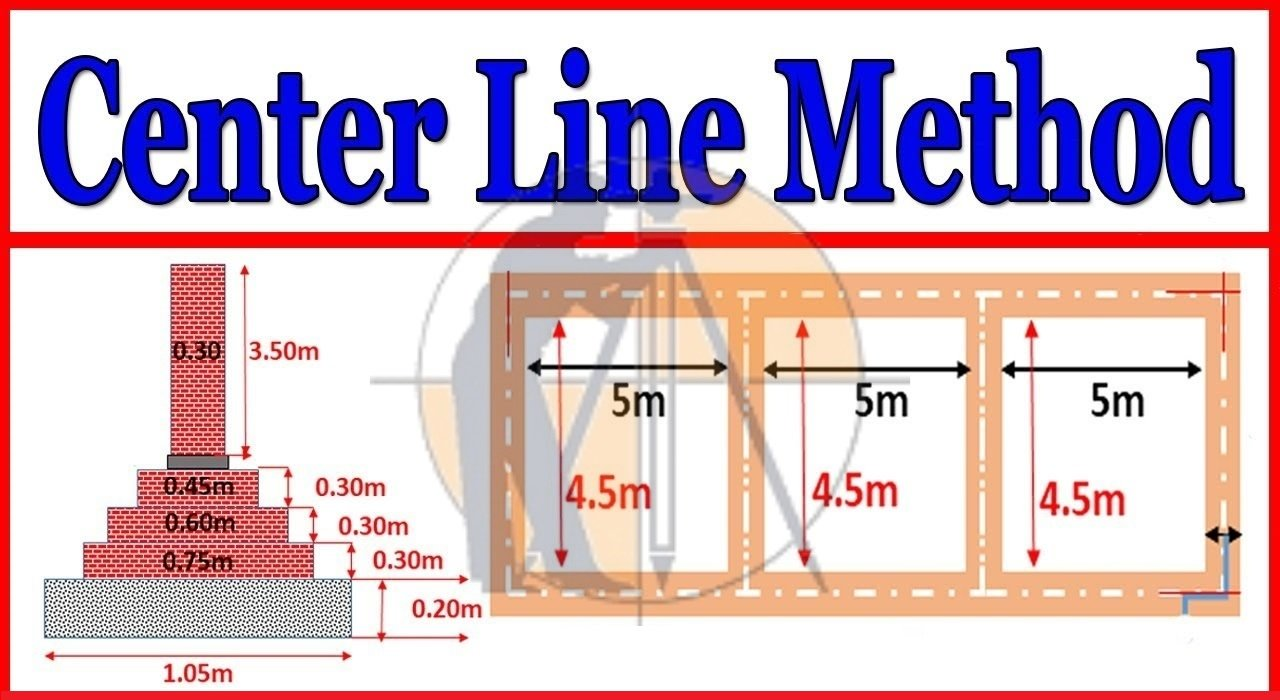## 1. Building Estimation By Center Line Method.

In this method we will calculate the sum total length of center lines of walls, long and short has to be found out. Find the total length of center lines of walls of the same type. Long & short have the same type of foundations and footings & they find the quantities by multiplying the total center length by the respective breadth and height.

In this method, the length will remain the same for excavations in the foundations for concrete in the foundations. For all footings & for the superstructure. (With slight difference when there are cross walls or the numbers of junctions). This method is quick but requires special attention & consideration at the junction, meeting points of cross walls, etc.

## 2. How to Calculate Center Line In Plan.

Center line length of the one wall = inner dimension of the room + Wall thickness

= Inner dimension of room + ½ x Wall thickness of one side + ½ x wall thickness of other.

• Centre line length of the horizontal wall

= 4 + 0.45 + 0.45

= 4.90 m

• #### Centre line length of vertical wall

= 3 + 0.45 + 0.45

= 3.90 m

= 2(4.90 + 3.90)

= 17.6 m

### Bar Bending Schedule for RCC Beam In Full Detail

#### Quantity of the Excavation for foundation.

Total length of center line of walls breath of trench x Depth of trench

= 17.6 x 0.9 x 1.2

= 19.01 m3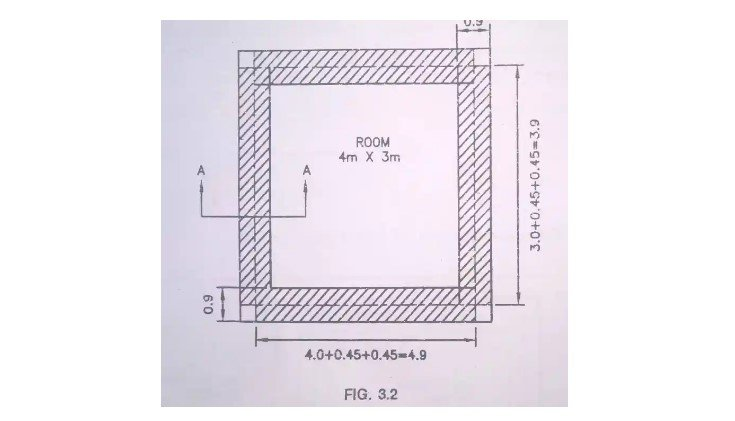For the rectangular, circular or polygonal buildings having no inter or cross walls, this method is quite simple but for buildings having a cross or partition walls, for every junctions of the partition or cross walls with main walls, a half breath of the respective item or footings is to be deducted from the total center length.

Net Centre line length – (½ x breath of wall x number of the junction)

Following Points in relation to the center line method should be noted.

### What Is Estimation and Costing

• The term junction is used to indicate the meeting points of cross walls or partition walls with main walls.
• For instance, if there is one cross wall there will be two junctions. At the corner of a building, no junction is formed.
• For rectangular building with no cross wall there will not be any junction & hence no deduction from the center line length will be required. It is fact that is certain portion taken twice is counter balanced by same amount portion left out at the corner.

• The breath indicates the width of item whose center line is being worked, for instance it will be the width of trench of excavations & foundation concrete. But for bricks masonry up to plinth or for superstructure will be equal to the corresponding thickness of the wall.

For a 2-room building with the inner cross wall, 2 junctions will be formed shown in fig.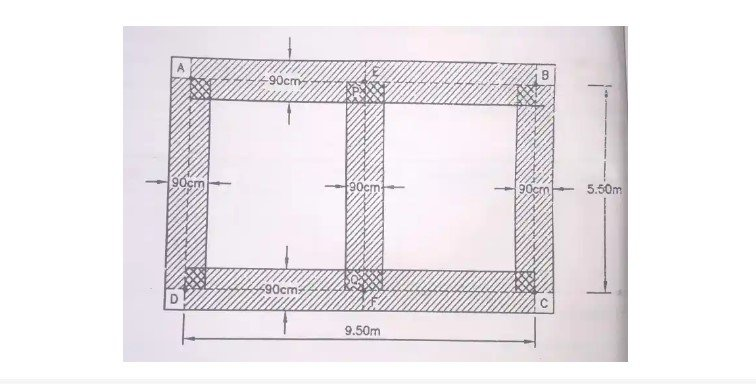### Total Centre Line length

= (2 x AB + 2 X BC + EF)

= (2 X 9.50 + 2 X 5.5 + 5.5)

If this center line length is multiplied by the width & depth, the quantity at junction P & Q will come twice, the quantity of each being equal to  ( ½ x width x Depth ).

Hence the quantity of these two junctions must be deducted to get the net center line length.

### Net Center Line Length

= (Total Centre line length – (width/ 2) x No. of junction)

= 35. 50 – (½ x 0.90) x 2

= 34. 60 m

## 3. Center Line Method of The Building Estimation Example.

Let’s take on example of a building plan & calculate its quantity by using the center line method of estimation.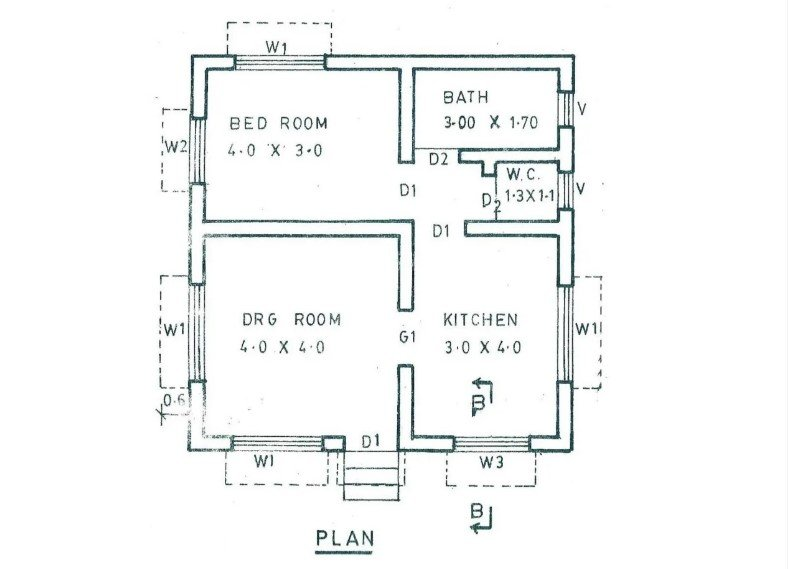### What Is Difference Between Pre-tension And Post-tension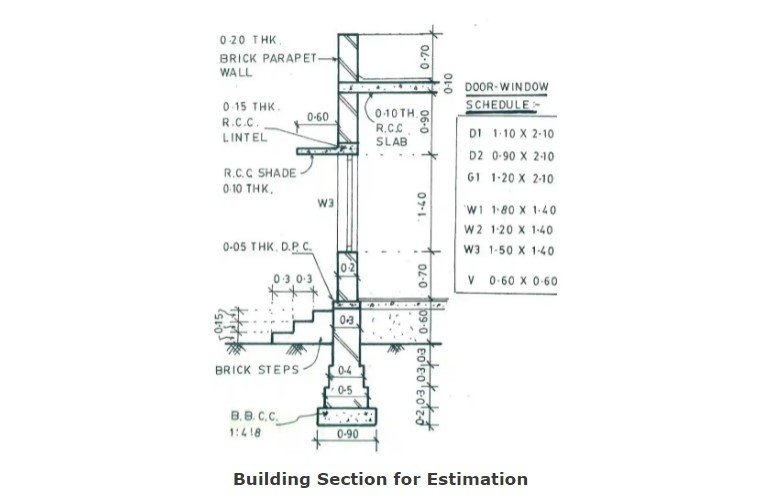### Building Estimation by Center Line Method Excel Sheet.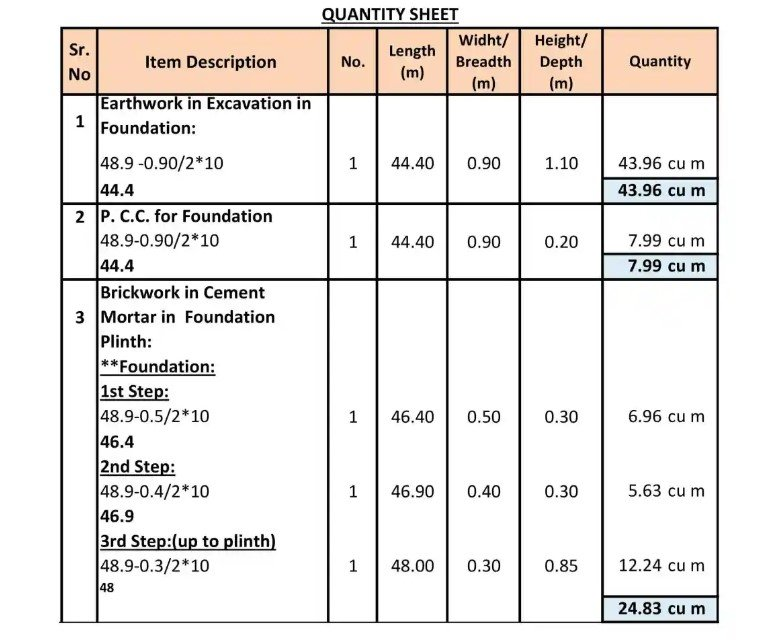2. ### How To Calculate The Cutting Length Of Circular Stirrup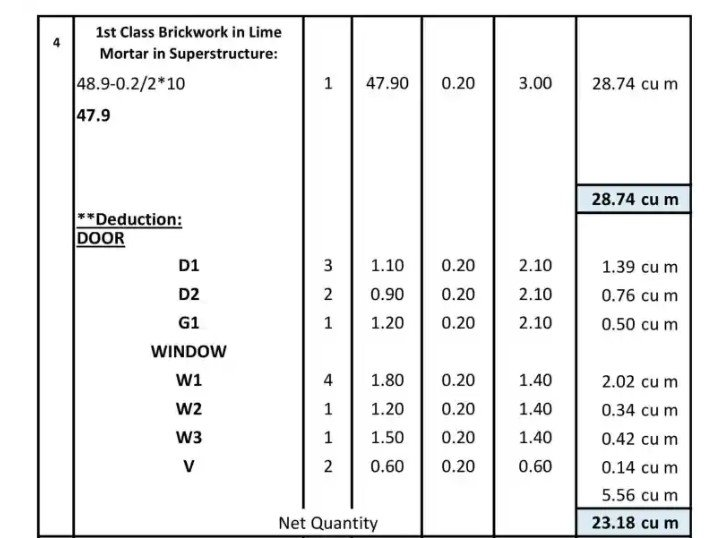### How to Calculate Cutting Length of Rectangular Stirrups And Circular Stirrups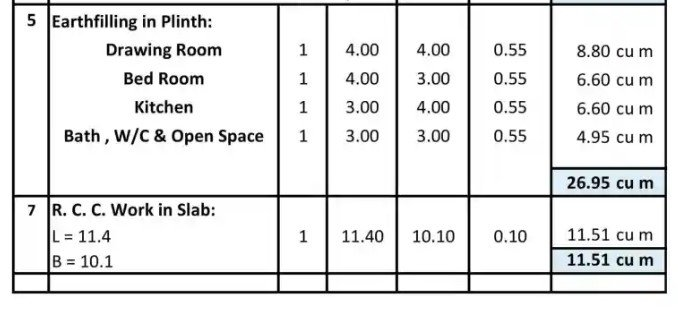## Other Post.

### THANKS.